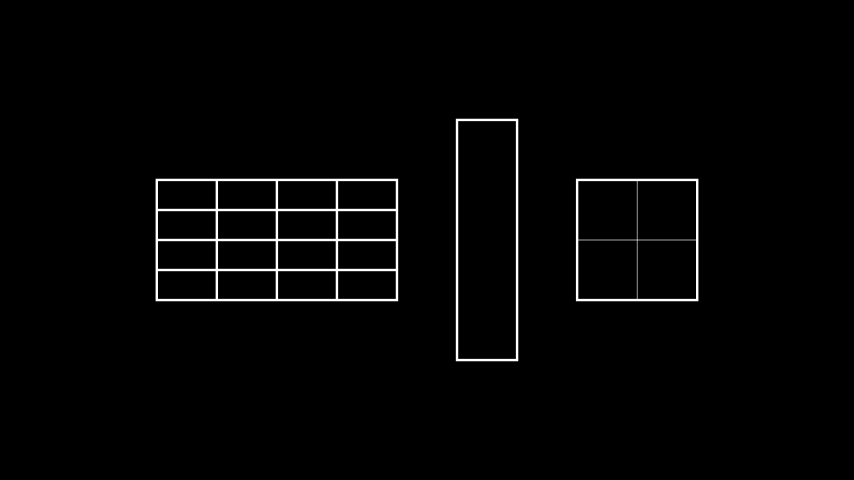# Rectangle#

Qualified name: `manim.mobject.geometry.polygram.Rectangle`

class Rectangle(color='#FFFFFF', height=2.0, width=4.0, grid_xstep=None, grid_ystep=None, mark_paths_closed=True, close_new_points=True, **kwargs)[source]#

Bases: `Polygon`

A quadrilateral with two sets of parallel sides.

Parameters
• color (Color) – The color of the rectangle.

• height (float) – The vertical height of the rectangle.

• width (float) – The horizontal width of the rectangle.

• grid_xstep (float | None) – Space between vertical grid lines.

• grid_ystep (float | None) – Space between horizontal grid lines.

• mark_paths_closed (bool) – No purpose.

• close_new_points (bool) – No purpose.

• kwargs – Additional arguments to be passed to `Polygon`

Examples

Example: RectangleExample```from manim import *

class RectangleExample(Scene):
def construct(self):
rect1 = Rectangle(width=4.0, height=2.0, grid_xstep=1.0, grid_ystep=0.5)
rect2 = Rectangle(width=1.0, height=4.0)

rects = Group(rect1,rect2).arrange(buff=1)
 `animate` Used to animate the application of any method of `self`. `animation_overrides` `color` `depth` The depth of the mobject. `fill_color` If there are multiple colors (for gradient) this returns the first one `height` The height of the mobject. `n_points_per_curve` `sheen_factor` `stroke_color` `width` The width of the mobject.